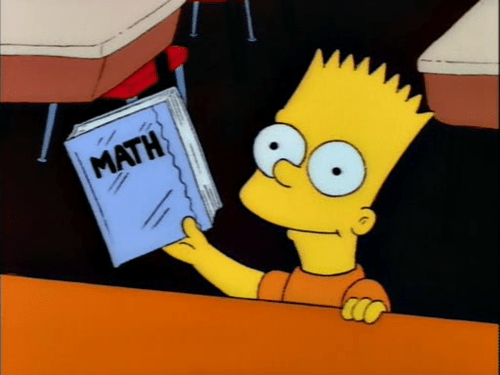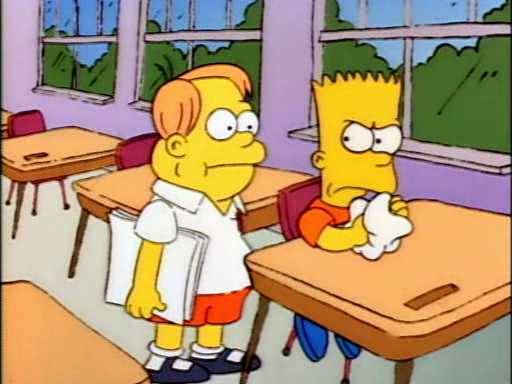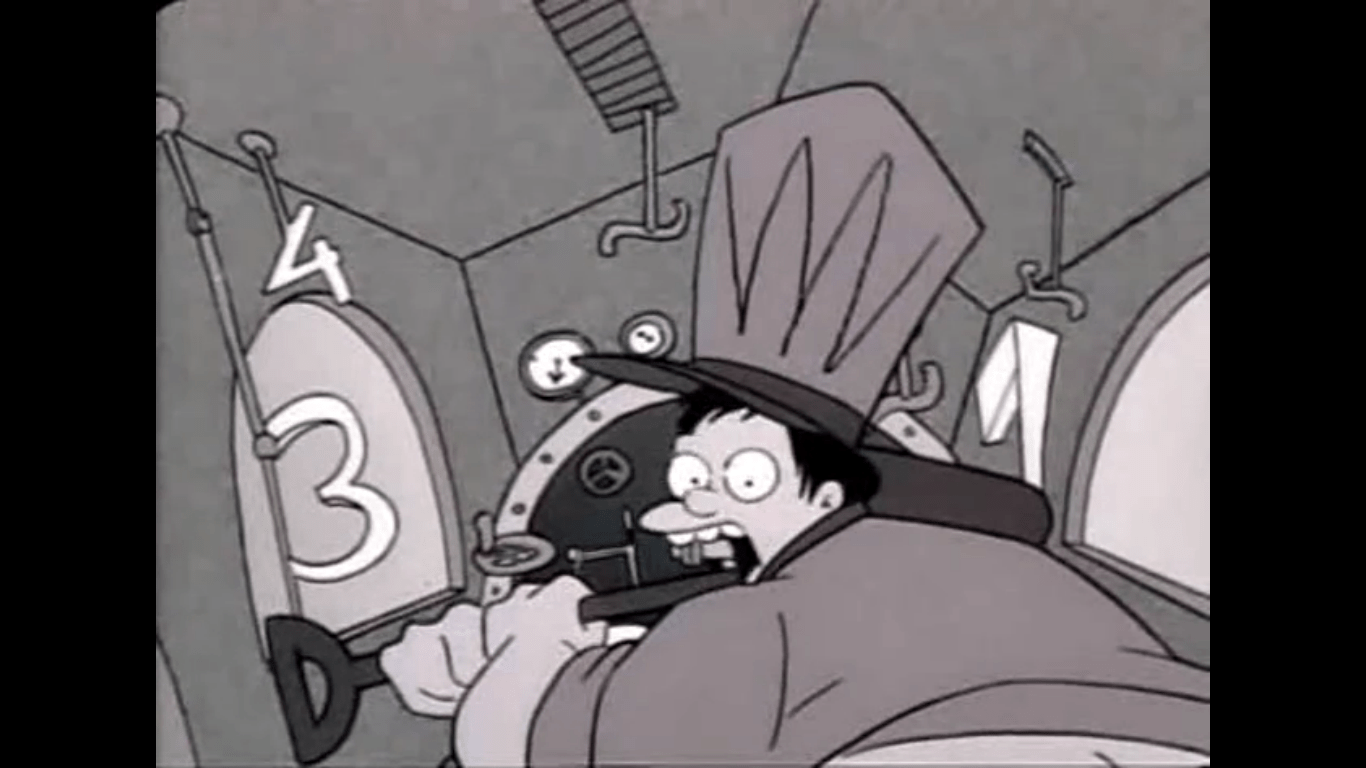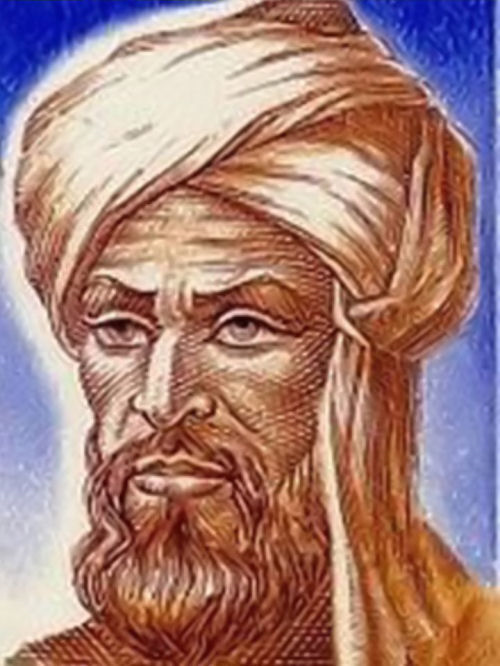# Super Safi’s Monday Morning Math Mayhem 20 – Bart’s Train Teasers

Morning Mathematical Monsters & Maniacs!

(Today’s post is sponsored by the letter “M”)

Hi, I’m Super Safi and you may remember me from such stats and strategy posts as Kwik-E-Mart Farming and the advanced losing-to-win Superheroes battle strategy.

Over the past 600+ episodes, The Simpsons has taken us on an amazing mathematical journey involving fractions, probability, Fermat’s last theorem, and hundreds of other aspects from the wonderful world off mathematics.

And what better way to start your week, then by discussing math Monday morning?With a new season of our Favourite Family just around the corner, we resume by going way back to season 1 and one of the very first scenes of the show in it’s regular timeslot.

Way back on January 14 1990, Bart the Genius (Season 01, Episode 02), became the first episode to use the famous opening sequence that we are now all too familiar with. During the episode, Edna Krabappel has the students complete an aptitude test.Bart doing his test starts reading:

“7:30am an express train traveling 60 miles per hour leaves Santa Fe bound for Phoenix, 520 miles away. At the same time, a local train traveling 30 miles an hour carrying 40 passengers leaves Phoenix bound for Santa Fe. It’s 8 cars long and always carries the same number of passengers in each car. An hour later, the number of passengers equal to half the number of minutes past the hour get off, but three times as many plus six get on. At the second stop, half the passengers plus two get off but twice as many get on as got on at the first stop.”So let’s look at some math questions that could come out of this paragraph:

Speed

Italian physicist Galileo Galilei is usually credited with being the first to measure speed. Galileo defined speed as the distance covered per unit of time. In equation form, that is   v   =   d   ÷   t   , where v is speed, d is distance, and t is time.Galileo Galilei

In the sentence “7:30am an express train traveling 60 miles per hour leaves Santa Fe bound for Phoenix, 520 miles away.”, we have v equal to 60 miles per hour and d of 520 miles. As we know two of the three variables, we can calculate the third. We just need to rearrange the equation to calculate t.

v   =   d   ÷   t   can be rearranged as   t   =   d   ÷   v

t   =   d   ÷   v   =   520 miles   ÷   60 miles per hour   =   8 hours and 40 minutes

So we have calculated that the express train will take 8 hours and 40 minutes to travel 520 miles from Santa Fe to Phoenix at a speed of 60 miles per hour.

We can likewise calculate for the second sentence “At the same time, a local train traveling 30 miles an hour carrying 40 passengers leaves Phoenix bound for Santa Fe.”

t   =   d   ÷   v   =   520 miles   ÷   30 miles per hour   =   17 hours and 20 minutes

So using the same formula, we have calculated that the local train will take 17 hours and 20 minutes to travel 520 miles from Santa Fe to Phoenix at a speed of 30 miles per hour.

Algebra

Algebra is the study of mathematical symbols and the rules for manipulating these symbols. The term comes from the book al-jabr by the 8th century Persian mathematician and astronomer al-Khwarizmi.al-Khwarizmi

Using algebra, we will try to solve how many passengers are on the local train in the remaining sentences “At the same time, a local train traveling 30 miles an hour carrying 40 passengers leaves Phoenix bound for Santa Fe. It’s 8 cars long and always carries the same number of passengers in each car. An hour later, the number of passengers equal to half the number of minutes past the hour get off, but three times as many plus six get on. At the second stop, half the passengers plus two get off but twice as many get on as got on at the first stop.”

So the local train has 40 passengers (p) and 8 cars (c). “It’s 8 cars long and always carries the same number of passengers in each car.” If each car carries the same number of passengers, then that number can be obtained by the equation p   ÷   c.

p   ÷   c    =    40   ÷   8   =   5   (as seen in Bart’s imagination)“An hour later, the number of passengers equal to half the number of minutes past the hour get off”. So if the train left at 7:30am, an hour later would be 8:30am; aka 30 minutes past the hour. So 15 passengers get off (30 ÷ 2). “but three times as many plus six get on”. So 51 get on (3 x 15 + 6)

p   =   40   –   15   +   51   =   76

So after departing from the first stop, the train now carries 76 passengers. (Now how each of the 8 cars have the same number of passengers is a mystery, but I digress.)

Now for those 76 passengers, “At the second stop, half the passengers plus two get off”. So 40 passengers get off (76 ÷ 2 + 2). “but twice as many get on as got on at the first stop”. So 102 get on (51 x 2).

p   =   76   –   40   +   102   =   138

So after departing from the second stop, the train now carries 138 passengers. (Now how each of the 8 cars have the same number of passengers is a mystery, but I digress.)

Now that we’ve completed a look at some of Bart’s aptitude test, we can look forward to the newest season of The Simpsons starting on Sunday. But for those of you burning for a little more math, another question that could arise from the above paragraph could ask what time and where do the express and local trains cross path?

Were you familiar with the speed equation? How much do you recall about algebra? Did you remember that it was Galileo and al-Khwarizmi that were the first to describe both? Sound off in the comments below. You know we love hearing from you.

P.S. Sorry for not posting this at 1:17pm today, but I might be enjoying 173mL of Arizona iced tea at that time.

### 13 responses to “Super Safi’s Monday Morning Math Mayhem 20 – Bart’s Train Teasers”

1.Cactus Pete

Of course, there are no trains between Santa Fe and Phoenix. Never have been.

2.NicoRox13

Let’s call the Phoenix bound train, Train P and the Santa Fe bound train, Train S.

Further, let’s assume that they leave at the same time and are on the same track with the same speeds as in the original question. Further, let’s assume that the local train, doe not

•NicoRox13

Sorry, let me continue…

Let’s assume the local train does not make stops but travels continuously at the rate given.

At the same time that the trains start, a super fast fly F starts at the front of Train P. It travels along the train track at 200 miles per hour. When it comes to Train S it immediately turns around and travel s back towards Train P. When it reaches Train P it once again turns around and continues in this fashion until it gets squashed between the two trains.

Let’s also assume that the fly F’s turn around time is negligible. That is, it does not lose speed at all.

Question. What is the total distance covered by the fly F?

•koolviruz

What is the total distance covered by the fly F? A= I life time…. “Assume” lets brake that down, ass + u + me = assume..

•sandytoes74

OH MY GOD STOP!!!!😱

•NicoRox13

I’m just getting started.

•sandytoes74

Then YOU can help my kids with their math homework.

•OsemAndy

•NicoRox13

Send them over, I’d be glad to help.

•sandytoes74

I’ll get them on Train P and ship them ur way…..

•NicoRox13

If you recall train P will be colliding with train S. Make sure your insurance is up to date.

•sandytoes74

Im a terrible mother😞

•NicoRox13